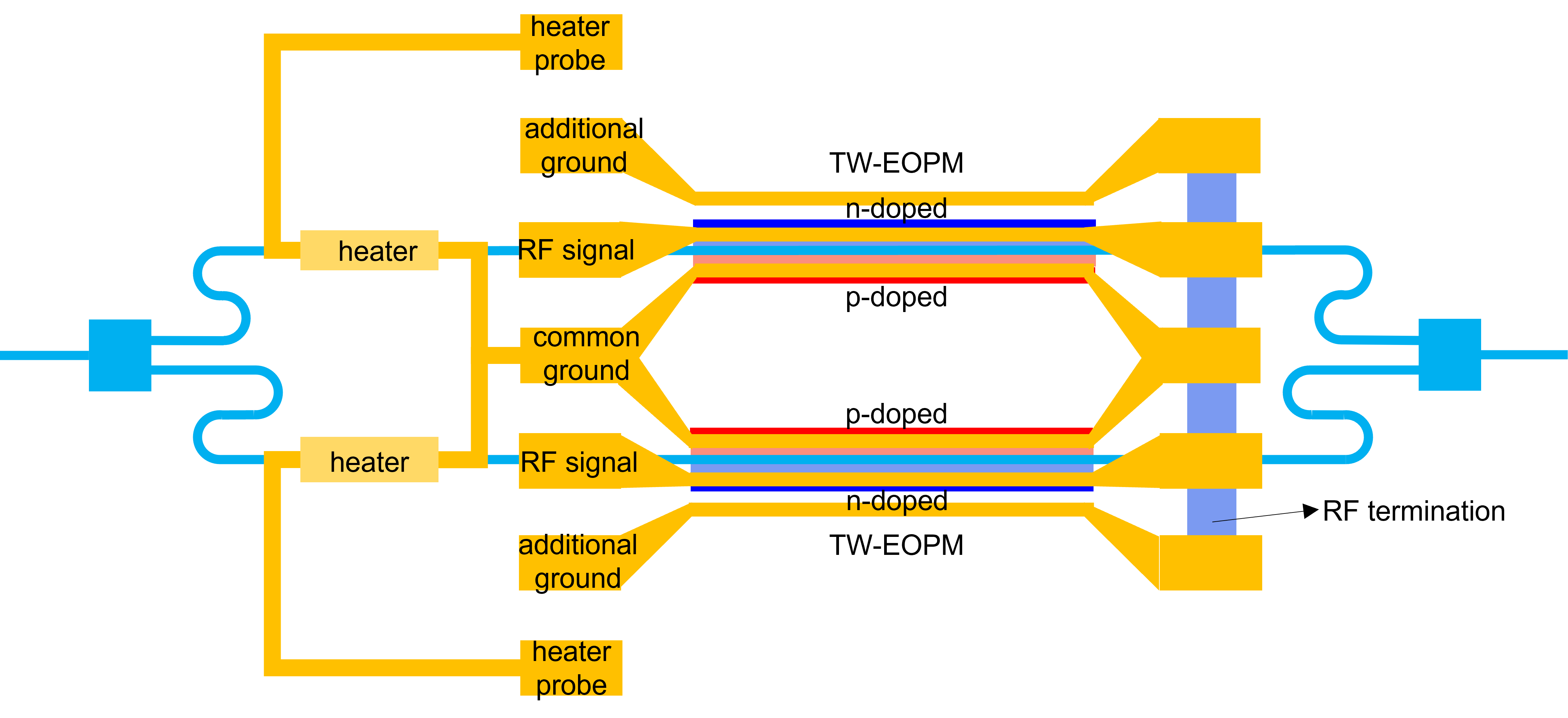# 1.4. Mach-Zehnder modulator (MZM)¶

As explained in the introduction, a Mach-Zehnder modulator is based on a Mach-Zehnder interferometer (MZI), which splits the light in two branches and then recombines them by interference. In each branch, a phase shifter is placed to control the relative phase shift of the light in the two branches. This allows us to modulate the phase in order to achieve constructive or destructive interference, which results in amplitude modulation.## 1.4.1. Layout¶

The layout of the MZM is mainly described by the properties of its sub-components, which are the phase shifter, the heater and the taper.

In addition, some other properties control how the modulator is connected electrically:

• rf_pitch
• rf_pad_length
• rf_pad_width
• rf_signal_width
• rf_ground_width

One particular point of interest is how we make sure that, under nominal conditions, a maximal extinction ratio is obtained by having a $$\pi / 2$$ length difference at center_wavelength.

The length difference between the two arms is obtained directly from the waveguide model at center_wavelength. It is implemented in the layout class of the MZM using a small U-bend in the waveguide.

## 1.4.2. Model and simulation recipes¶

The circuit model of the MZM is a hierarchical model that is built on the individual models of the following components:

• Phase shifter
• Splitter
• Taper
• Heater
• Waveguides

The simulation recipes can be used to simulate the modulator, check the modulation efficiency and explore different compromises that can be made when designing this component.

Check out the following examples:

Exercise 1: Explore the design parameters

1. Copy si_fab/ipkiss/si_fab/components/modulator/mzm/doc/example_mzm_layout.py.
2. Explore the parameter space provided and inspect the layout in KLayout.
3. Check the unbalance of the two arms.

Exercise 2 - Modulation efficiency: varying the length of the phase shifter

1. Copy si_fab/ipkiss/si_fab/components/modulator/mzm/doc/example_mzm_sweep_length.py.
2. What is the maximum extinction ratio you can obtain with a PRBS signal at 5 Gbit/s?
3. Add noise to the modulation signal and check the effect.
4. Add noise to optical signal and check the effect.

Exercise 3 - Tuning: varying the heater length and voltage

1. Copy si_fab/ipkiss/si_fab/components/modulator/mzm/doc/example_mzm_sweep_heater.py.
2. Change the metal width of the heater. What is the effect?
3. Change the length of the heater. What is the effect?